Next: Two-State System Up: Probability Theory Previous: What is Probability?

# Combining Probabilities

Consider two distinct possible outcomes,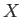and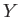, of an observation made on the system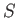, with probabilities of occurrence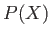and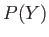, respectively. Let us determine the probability of obtaining either the outcomeor the outcome, which we shall denote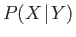. From the basic definition of probability,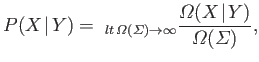(2.2)

where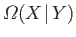is the number of systems in the ensemble that exhibit either the outcomeor the outcome. It is clear that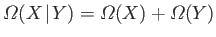(2.3)

if the outcomesandare mutually exclusive (which must be the case if they are two distinct outcomes). Thus,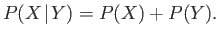(2.4)

We conclude that the probability of obtaining either the outcomeor the outcomeis the sum of the individual probabilities ofand. For instance, with a six-sided die, the probability of throwing any particular number (one to six) is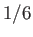, because all of the possible outcomes are considered to be equally likely. It follows, from the previous discussion, that the probability of throwing either a one or a two is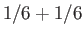, which equals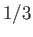.

Let us denote all of the, say, possible outcomes of an observation made on the systemby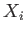, where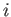runs from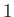to. Let us determine the probability of obtaining any of these outcomes. This quantity is clearly unity, from the basic definition of probability, because every one of the systems in the ensemble must exhibit one of the possible outcomes. However, this quantity is also equal to the sum of the probabilities of all the individual outcomes, by Equation (2.4), so we conclude that this sum is equal to unity. Thus,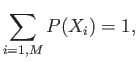(2.5)

which is called the normalization condition, and must be satisfied by any complete set of probabilities. This condition is equivalent to the self-evident statement that an observation of a system must definitely result in one of its possible outcomes.

There is another way in which we can combine probabilities. Suppose that we make an observation on a state picked at random from the ensemble, and then pick a second state, completely independently, and make another observation. Here, we are assuming that the first observation does not influence the second observation in any way. In other words, the two observations are statistically independent. Let us determine the probability of obtaining the outcomein the first state and the outcomein the second state, which we shall denote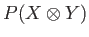. In order to determine this probability, we have to form an ensemble of all of the possible pairs of states that we could choose from the ensemble,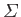. Let us denote this ensemble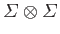. It is obvious that the number of pairs of states in this new ensemble is just the square of the number of states in the original ensemble, so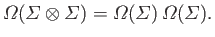(2.6)

It is also fairly obvious that the number of pairs of states in the ensemblethat exhibit the outcomein the first state, andin the second state, is just the product of the number of states that exhibit the outcome, and the number of states that exhibit the outcome, in the original ensemble. Hence,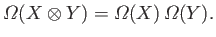(2.7)

It follows from the basic definition of probability that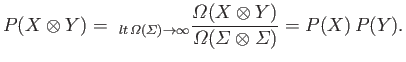(2.8)

Thus, the probability of obtaining the outcomesandin two statistically independent observations is the product of the individual probabilities ofand. For instance, the probability of throwing a one and then a two on a six-sided die is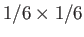, which equals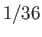.Next: Two-State System Up: Probability Theory Previous: What is Probability?
Richard Fitzpatrick 2016-01-25